Prev: List of Tables Up: Dissertation W. Pyka Next: List of Abbreviations

# List of Symbols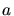,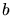,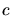Diameters of the structuring element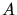,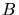,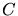Bleachable absorption coefficients of Dill's `ABC'-model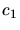,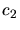Reaction rate constants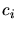Concentration of species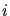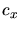Geometric complexity exponent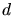Averaging distance for calculating surface normals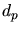Particle diameter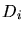Diffusion coefficient of species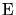Ion enhancement factor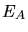Activation energy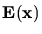Complex-valued phasor of time-harmonic electric field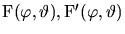Angular distribution functions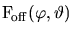Angular distribution function for off center position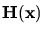Complex-valued phasor of time-harmonic magnetic field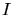Electrical current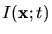Intensity distribution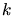Boltzmann number, 1.381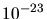J/K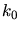Characteristic parameter of lithography processes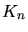Knudsen number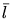Mean free path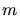Fitting paramter for particle distribution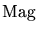Magnitude of electrical field component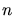Number of cells in one dimension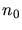Refractive Index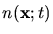Complex-valued refractive indexFraction of convex corner surface cells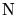Normalizing factor for distribution functions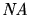Numerical aperture of a lens or a lens system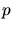Pressure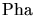Phase of electrical field componentLocal etch or deposition rate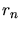Rate resolved by cells size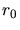Nominal etch or deposition rate for flat wafer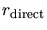Etch or deposition contribution from directly incident particlesGas constant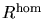,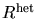Homogeneous and heterogeneous reaction rate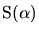Angular dependent etching yield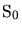Etching yield for normal incidence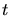Time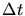Time-step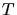Temperature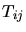Reflection transformation matrix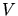Voltage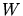Lithographic resolution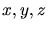Position along x-, y-, and z-axis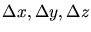Differerence in position in x-, y-, and z-Axis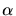AngleAngular step width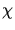Reciprocal relative permittivity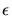Energy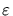Permittivity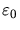Vacuum permittivity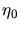Free-space resistance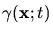Light sensitive photoresist compound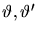Azimuthal angleAzimuthal direction of the sputter target center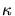Dielectric constant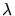Actinic wavelength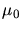Vacuum permeability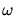Angular frequency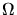Solid angle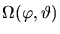Visibility function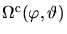Complementary visibility function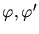Polar angle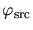Polar direction of the sputter target center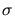Sticking coefficient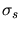Standard deviationPrev: List of Tables Up: Dissertation W. Pyka Next: List of Abbreviations

W. Pyka: Feature Scale Modeling for Etching and Deposition Processes in Semiconductor Manufacturing